Work, Energy, and Energy Resources

# Kinetic Energy and the Work-Energy Theorem

OpenStaxCollege

### Learning Objectives

• Explain work as a transfer of energy and net work as the work done by the net force.
• Explain and apply the work-energy theorem.

# Work Transfers Energy

What happens to the work done on a system? Energy is transferred into the system, but in what form? Does it remain in the system or move on? The answers depend on the situation. For example, if the lawn mower in [link](a) is pushed just hard enough to keep it going at a constant speed, then energy put into the mower by the person is removed continuously by friction, and eventually leaves the system in the form of heat transfer. In contrast, work done on the briefcase by the person carrying it up stairs in [link](d) is stored in the briefcase-Earth system and can be recovered at any time, as shown in [link](e). In fact, the building of the pyramids in ancient Egypt is an example of storing energy in a system by doing work on the system. Some of the energy imparted to the stone blocks in lifting them during construction of the pyramids remains in the stone-Earth system and has the potential to do work.

In this section we begin the study of various types of work and forms of energy. We will find that some types of work leave the energy of a system constant, for example, whereas others change the system in some way, such as making it move. We will also develop definitions of important forms of energy, such as the energy of motion.

# Net Work and the Work-Energy Theorem

We know from the study of Newton’s laws in Dynamics: Force and Newton’s Laws of Motion that net force causes acceleration. We will see in this section that work done by the net force gives a system energy of motion, and in the process we will also find an expression for the energy of motion.

Let us start by considering the total, or net, work done on a system. Net work is defined to be the sum of work done by all external forces—that is, net work is the work done by the net external force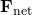. In equation form, this is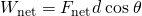where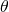is the angle between the force vector and the displacement vector.

[link](a) shows a graph of force versus displacement for the component of the force in the direction of the displacement—that is, an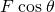vs.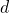graph. In this case,is constant. You can see that the area under the graph is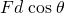, or the work done. [link](b) shows a more general process where the force varies. The area under the curve is divided into strips, each having an average force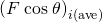. The work done is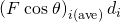for each strip, and the total work done is the sum of the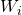. Thus the total work done is the total area under the curve, a useful property to which we shall refer later.

(a) A graph ofvs., whenis constant. The area under the curve represents the work done by the force. (b) A graph ofvs.in which the force varies. The work done for each interval is the area of each strip; thus, the total area under the curve equals the total work done.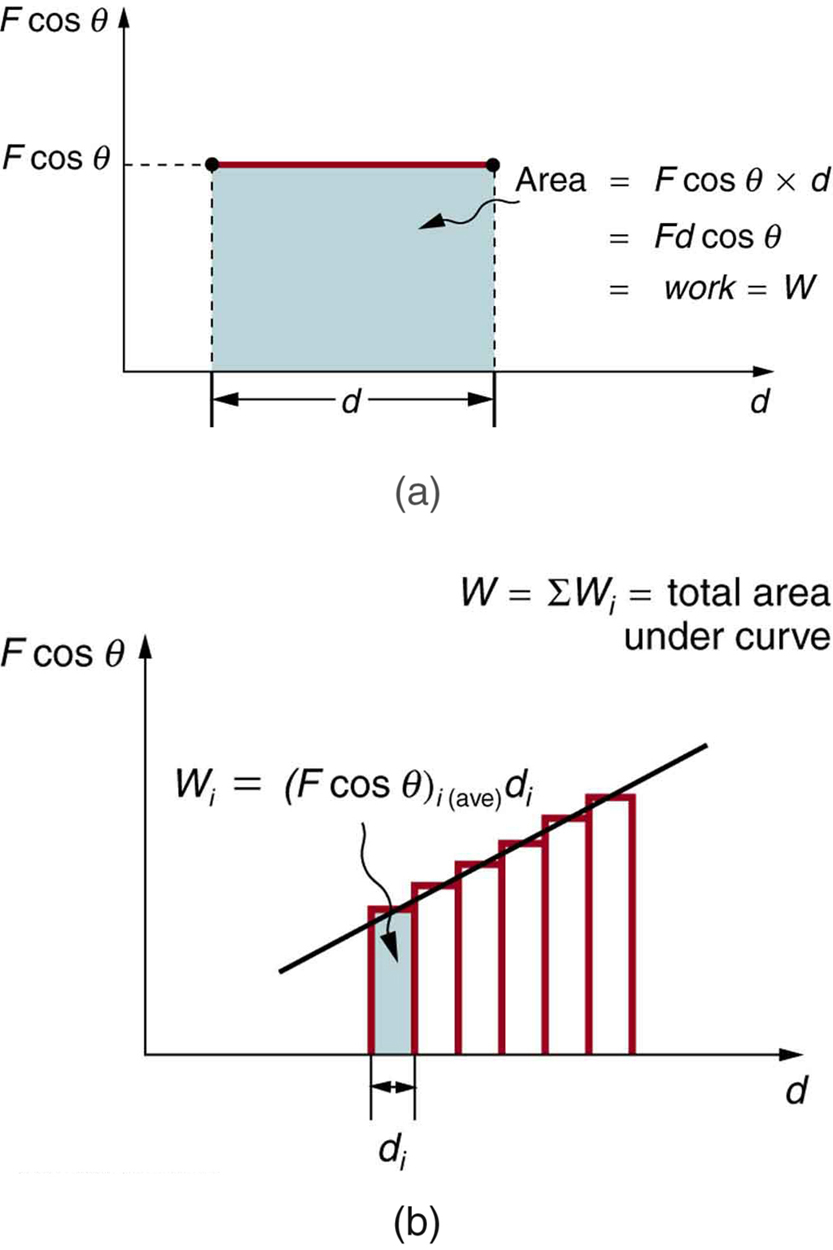Net work will be simpler to examine if we consider a one-dimensional situation where a force is used to accelerate an object in a direction parallel to its initial velocity. Such a situation occurs for the package on the roller belt conveyor system shown in [link].

A package on a roller belt is pushed horizontally through a distance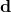.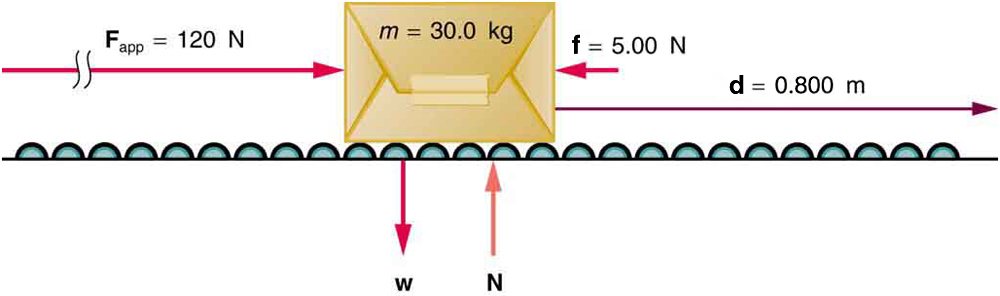The force of gravity and the normal force acting on the package are perpendicular to the displacement and do no work. Moreover, they are also equal in magnitude and opposite in direction so they cancel in calculating the net force. The net force arises solely from the horizontal applied force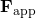and the horizontal friction force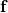. Thus, as expected, the net force is parallel to the displacement, so that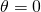and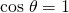, and the net work is given by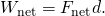The effect of the net forceis to accelerate the package from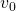to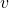. The kinetic energy of the package increases, indicating that the net work done on the system is positive. (See [link].) By using Newton’s second law, and doing some algebra, we can reach an interesting conclusion. Substituting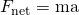from Newton’s second law gives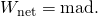To get a relationship between net work and the speed given to a system by the net force acting on it, we take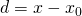and use the equation studied in Motion Equations for Constant Acceleration in One Dimension for the change in speed over a distanceif the acceleration has the constant value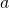; namely,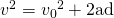(note thatappears in the expression for the net work). Solving for acceleration gives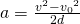. Whenis substituted into the preceding expression for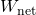, we obtain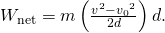Thecancels, and we rearrange this to obtain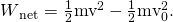This expression is called the work-energy theorem, and it actually applies in general (even for forces that vary in direction and magnitude), although we have derived it for the special case of a constant force parallel to the displacement. The theorem implies that the net work on a system equals the change in the quantity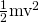. This quantity is our first example of a form of energy.

The Work-Energy Theorem

The net work on a system equals the change in the quantity.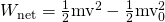The quantityin the work-energy theorem is defined to be the translational kinetic energy (KE) of a mass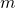moving at a speed. (Translational kinetic energy is distinct from rotational kinetic energy, which is considered later.) In equation form, the translational kinetic energy,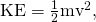is the energy associated with translational motion. Kinetic energy is a form of energy associated with the motion of a particle, single body, or system of objects moving together.

We are aware that it takes energy to get an object, like a car or the package in [link], up to speed, but it may be a bit surprising that kinetic energy is proportional to speed squared. This proportionality means, for example, that a car traveling at 100 km/h has four times the kinetic energy it has at 50 km/h, helping to explain why high-speed collisions are so devastating. We will now consider a series of examples to illustrate various aspects of work and energy.

Calculating the Kinetic Energy of a Package

Suppose a 30.0-kg package on the roller belt conveyor system in [link] is moving at 0.500 m/s. What is its kinetic energy?

Strategy

Because the massand speedare given, the kinetic energy can be calculated from its definition as given in the equation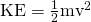.

Solution

The kinetic energy is given by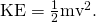Entering known values gives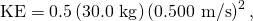which yields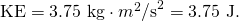Discussion

Note that the unit of kinetic energy is the joule, the same as the unit of work, as mentioned when work was first defined. It is also interesting that, although this is a fairly massive package, its kinetic energy is not large at this relatively low speed. This fact is consistent with the observation that people can move packages like this without exhausting themselves.

Determining the Work to Accelerate a Package

Suppose that you push on the 30.0-kg package in [link] with a constant force of 120 N through a distance of 0.800 m, and that the opposing friction force averages 5.00 N.

(a) Calculate the net work done on the package. (b) Solve the same problem as in part (a), this time by finding the work done by each force that contributes to the net force.

Strategy and Concept for (a)

This is a motion in one dimension problem, because the downward force (from the weight of the package) and the normal force have equal magnitude and opposite direction, so that they cancel in calculating the net force, while the applied force, friction, and the displacement are all horizontal. (See [link].) As expected, the net work is the net force times distance.

Solution for (a)

The net force is the push force minus friction, or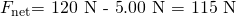. Thus the net work is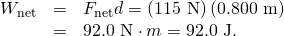Discussion for (a)

This value is the net work done on the package. The person actually does more work than this, because friction opposes the motion. Friction does negative work and removes some of the energy the person expends and converts it to thermal energy. The net work equals the sum of the work done by each individual force.

Strategy and Concept for (b)

The forces acting on the package are gravity, the normal force, the force of friction, and the applied force. The normal force and force of gravity are each perpendicular to the displacement, and therefore do no work.

Solution for (b)

The applied force does work.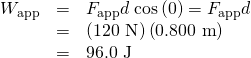The friction force and displacement are in opposite directions, so that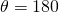, and the work done by friction is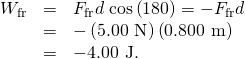So the amounts of work done by gravity, by the normal force, by the applied force, and by friction are, respectively,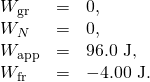The total work done as the sum of the work done by each force is then seen to be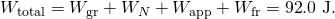Discussion for (b)

The calculated total work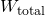as the sum of the work by each force agrees, as expected, with the workdone by the net force. The work done by a collection of forces acting on an object can be calculated by either approach.

Determining Speed from Work and Energy

Find the speed of the package in [link] at the end of the push, using work and energy concepts.

Strategy

Here the work-energy theorem can be used, because we have just calculated the net work,, and the initial kinetic energy,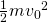. These calculations allow us to find the final kinetic energy,, and thus the final speed.

Solution

The work-energy theorem in equation form is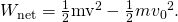Solving forgives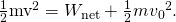Thus,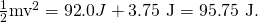Solving for the final speed as requested and entering known values gives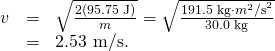Discussion

Using work and energy, we not only arrive at an answer, we see that the final kinetic energy is the sum of the initial kinetic energy and the net work done on the package. This means that the work indeed adds to the energy of the package.

Work and Energy Can Reveal Distance, Too

How far does the package in [link] coast after the push, assuming friction remains constant? Use work and energy considerations.

Strategy

We know that once the person stops pushing, friction will bring the package to rest. In terms of energy, friction does negative work until it has removed all of the package’s kinetic energy. The work done by friction is the force of friction times the distance traveled times the cosine of the angle between the friction force and displacement; hence, this gives us a way of finding the distance traveled after the person stops pushing.

Solution

The normal force and force of gravity cancel in calculating the net force. The horizontal friction force is then the net force, and it acts opposite to the displacement, so. To reduce the kinetic energy of the package to zero, the work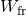by friction must be minus the kinetic energy that the package started with plus what the package accumulated due to the pushing. Thus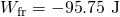. Furthermore,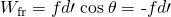, where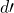is the distance it takes to stop. Thus,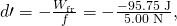and so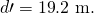Discussion

This is a reasonable distance for a package to coast on a relatively friction-free conveyor system. Note that the work done by friction is negative (the force is in the opposite direction of motion), so it removes the kinetic energy.

Some of the examples in this section can be solved without considering energy, but at the expense of missing out on gaining insights about what work and energy are doing in this situation. On the whole, solutions involving energy are generally shorter and easier than those using kinematics and dynamics alone.

# Section Summary

• The net workis the work done by the net force acting on an object.
• Work done on an object transfers energy to the object.
• The translational kinetic energy of an object of massmoving at speedis.
• The work-energy theorem states that the net workon a system changes its kinetic energy,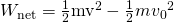.

# Conceptual Questions

The person in [link] does work on the lawn mower. Under what conditions would the mower gain energy? Under what conditions would it lose energy?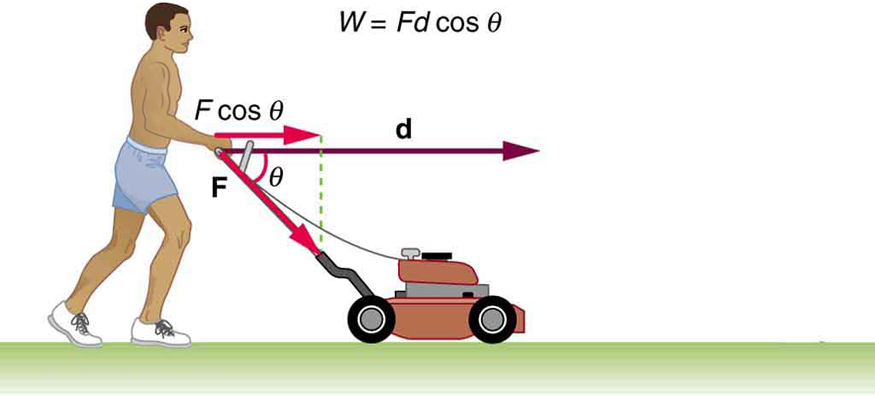Work done on a system puts energy into it. Work done by a system removes energy from it. Give an example for each statement.

When solving for speed in [link], we kept only the positive root. Why?

# Problems & Exercises

Compare the kinetic energy of a 20,000-kg truck moving at 110 km/h with that of an 80.0-kg astronaut in orbit moving at 27,500 km/h.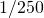(a) How fast must a 3000-kg elephant move to have the same kinetic energy as a 65.0-kg sprinter running at 10.0 m/s? (b) Discuss how the larger energies needed for the movement of larger animals would relate to metabolic rates.

Confirm the value given for the kinetic energy of an aircraft carrier in [link]. You will need to look up the definition of a nautical mile (1 knot = 1 nautical mile/h).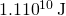(a) Calculate the force needed to bring a 950-kg car to rest from a speed of 90.0 km/h in a distance of 120 m (a fairly typical distance for a non-panic stop). (b) Suppose instead the car hits a concrete abutment at full speed and is brought to a stop in 2.00 m. Calculate the force exerted on the car and compare it with the force found in part (a).

A car’s bumper is designed to withstand a 4.0-km/h (1.1-m/s) collision with an immovable object without damage to the body of the car. The bumper cushions the shock by absorbing the force over a distance. Calculate the magnitude of the average force on a bumper that collapses 0.200 m while bringing a 900-kg car to rest from an initial speed of 1.1 m/s.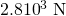Boxing gloves are padded to lessen the force of a blow. (a) Calculate the force exerted by a boxing glove on an opponent’s face, if the glove and face compress 7.50 cm during a blow in which the 7.00-kg arm and glove are brought to rest from an initial speed of 10.0 m/s. (b) Calculate the force exerted by an identical blow in the gory old days when no gloves were used and the knuckles and face would compress only 2.00 cm. (c) Discuss the magnitude of the force with glove on. Does it seem high enough to cause damage even though it is lower than the force with no glove?

Using energy considerations, calculate the average force a 60.0-kg sprinter exerts backward on the track to accelerate from 2.00 to 8.00 m/s in a distance of 25.0 m, if he encounters a headwind that exerts an average force of 30.0 N against him.

102 N

## Glossary

net work
work done by the net force, or vector sum of all the forces, acting on an object
work-energy theorem
the result, based on Newton’s laws, that the net work done on an object is equal to its change in kinetic energy
kinetic energy
the energy an object has by reason of its motion, equal tofor the translational (i.e., non-rotational) motion of an object of massmoving at speed